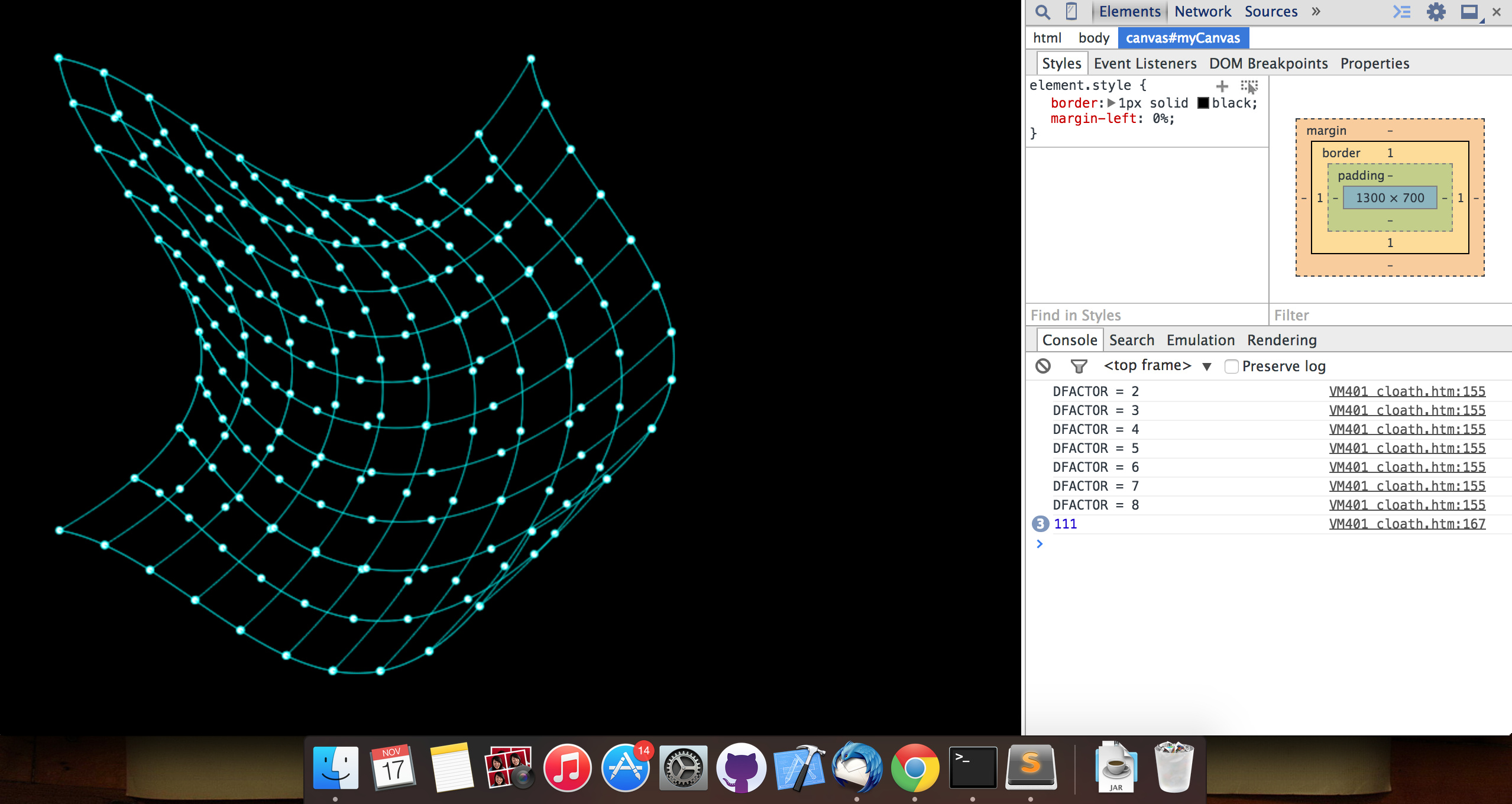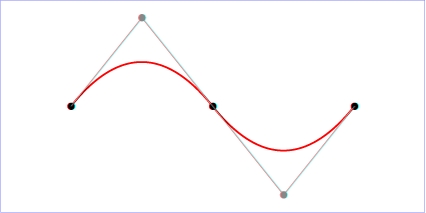### This post covers

• What’s a bezier equation?
• Creating a 3d surface patch using Bezier curves
• Making it interactiveScreenshot of experiment

### So what’s a Bezier curve?

Its a cubic parametric curve used to draw smooth curves, we can draw a bezier curve using two endpoints and two control points. It looks something like:Bezier Curve - Wikipedia

So how to draw the surface using multiple bezier curves:

### Well there are multiple ways, here’s what I did

• Drew my first Bezier curve (The top one) and kept track of 10 equidistant points on that curve say HP.
• Drew first vertical Bezier curve (The left one) and kept track of 10 equidistant points on that curve say VP.
• Now for each point in HP draw a vertical bezier with same control points. Similarly for all points in VP draw horizontal Bezier curves with same control points.
• In the meanwhile you can draw points at equidistant points on each bezier curve.
• The code looks something like:

How to make it interactive: Simply change the control points according to input (mouse move, key press in my case)

Complete code available at my github repo: My-Playground/inside-the-mesh